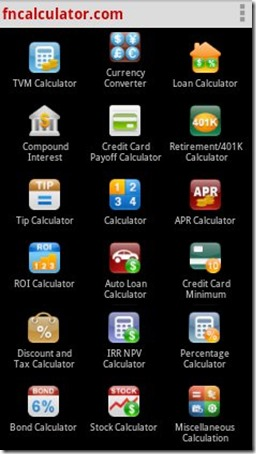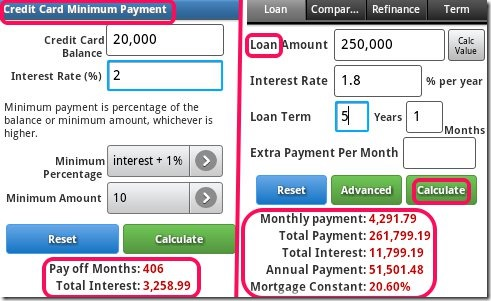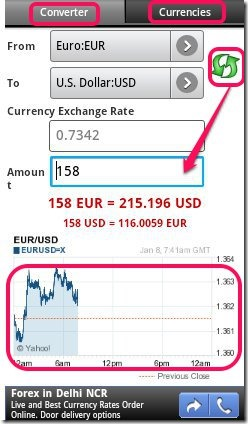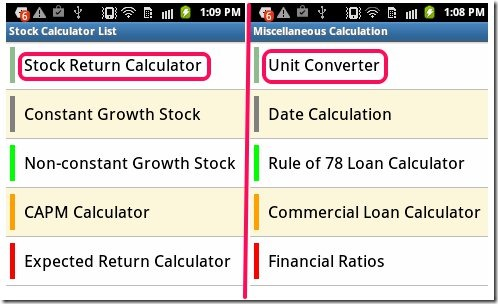Editor Ratings:
User Ratings:
[Total: 0 Average: 0]

Financial Calculators is an Android Calculator app that allows you to do any type of calculation. It consists of 18 Calculators. Each calculator has sub-forms. You can calculate anything from simple mathematical calculations to compound interest, loan payment, percentage, credit card minimum amount, return on investment, stock returns, discount and tax, etc.

This free app is a really unique and useful Android calculator that you can keep with you all the time and use to do almost all types of calculations without the need of searching for help online.## Key Features of Financial Calculators App:

• Simple to use Interface.
• More than 18 types of calculators in one app.
• Only Currency Converter requires internet access.
• No need of internet to use other Calculators.
• Look of the app can be customized.
• You can select the calculators that you want to see.
• Can be used on phone as well as on tablet.
• Easy to use calculators for all necessary calculation work.

If you are looking for an Expense Manager for Android then try iExpense Manager.## Using this free Android Calculator App:

You can download Financial Calculators using the link or QR Code given at the end of this review. Once the app is installed, launch it by tapping on its icon from the apps menu in your Android device. The app will show you a list of all the calculators. You can change to grid view from the setting as also select which calculators you want to see when you open the app.

Tap the icon of the calculator that you want to use and calculate whatever you require with the help of the calculators given in the app. These are as follows:

• TVM Calculator: It calculates time value of money.
• Currency Converter: You can check currency rates of almost all currencies of the world.• Loan Calculator: You can calculate loan payment, check comparisons, do refinance calculations, and calculate term required to pay the loan.
• Compound Interest Calculator: Using this calculator you can calculate compound interest.
• Credit Card Payoff Calculator: Calculate how much money you need to pay for credit card using this calculator.
• Retirement/401K Calculator: Calculator for employee savings plan for retirement.
• Tip Calculator: You can calculate how much tip you need to pay with this calculator.
• Regular Calculator: This calculator allows you to solve simple mathematical operations.
• APR Calculator: Calculate Annual Percentage rate with the help of this calculator.
• ROI Calculator: You can do calculations to know the Return on Investment using this calculator.
• Auto Loan Calculator: Calculate auto loan and Auto lease payments using this calculator.
• Credit Card Minimum Payment Calculator: Want to know the minimum amount you need to pay for your credit card/ then, use this calculator.
• Discount and Tax Calculator: This calculator can help you calculate discounts and tax.
• IRR NPV Calculator: Internal Rate of Return and Net Present Value can be calculated using this type of calculator.
• Percentage Calculator: Do you get confused when somebody asks what percentage of x is y? Now, solve all percentage woes with this calculator.
• Bond Calculator: Do all calculations related to bonds.
• Stock Calculator: It consists of 5 calculators for Stock Returns, Constant Growth Stock, Non-Constant Growth Stock, CAPM and Expected Returns.
• Miscellaneous Calculations: It consists of unit converter, date calculator, financial ratios etc..Also check  Total Calculator for Android, an Android calculator app reviewed on our website.

## My Verdict:

In my opinion, Financial Calculators is a very useful free app. It is an all in one Android calculator app that can be used to do most of the calculations a person could need to do.

Get Financial Calculators app for Android here.

You can also use the QR Code given below to get the app directly on your Android device.